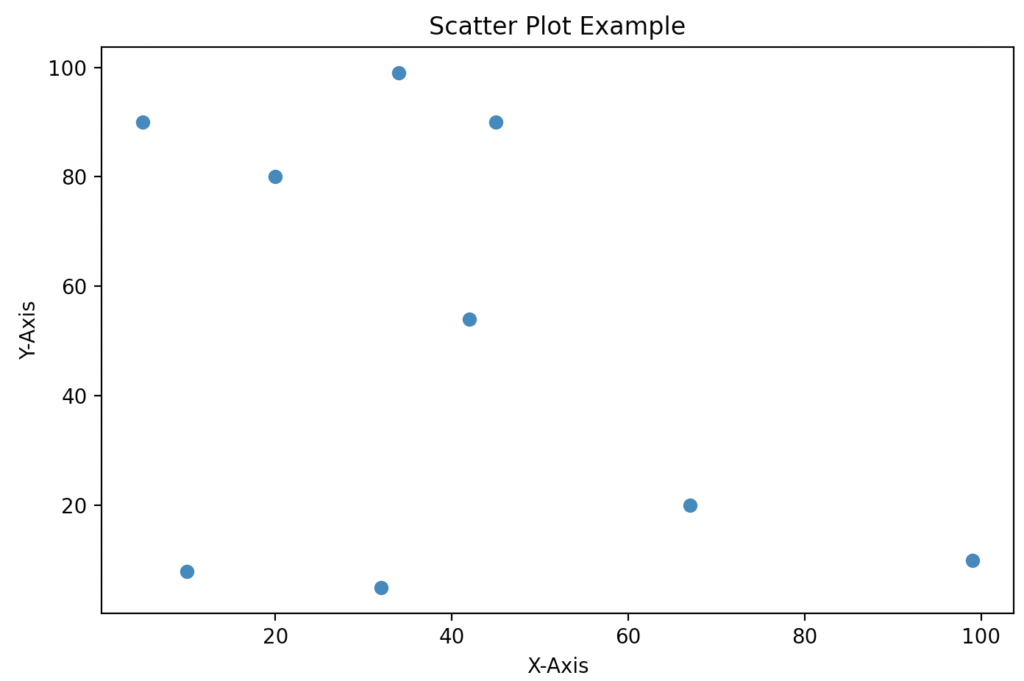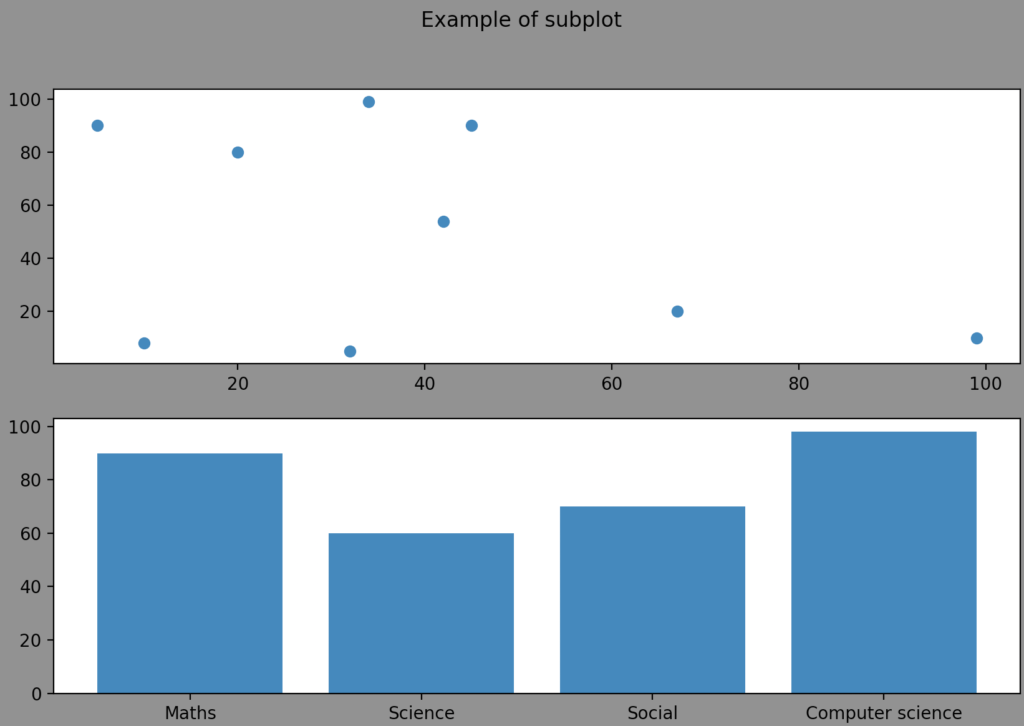# Matplotlib figure: The Complete Guide

The figure() in Python is a pyplot module of the matplotlib library used to create a new figure.

## Matplotlib figure

Matplotlib figure() is a library function used to create a new figure or activate an existing figure.

### Syntax

``````matplotlib.pyplot.figure(num=None, figsize=None, dpi=None, facecolor=None, edgecolor=None,
frameon=True, FigureClass=<class 'matplotlib.figure.Figure'>,
clear=False, **kwargs) ``````

### Arguments

The matplotlib.pyplot.figure() function has some optional arguments as parameter:

1. num: This argument takes string or integer values. This is the unique identifier provided for each image. If this unique identifier already exists, the existing figure will be activated.
2. figsize: This describes the figure size of the created image. The size of the image is passed in this argument. It takes two floating point values as values. The width and inches are passed in this argument.
3. dpi: This dpi stands for dots per inch. This argument takes floating numbers as values. This argument is used to describe the resolution in which the figure is needed to be created.
4. facecolor: The facecolor of the image is passed in this argument. This is the background color of the figure.
5. edgecolor: The edge color of the figure is passed in this argument.
6. frameon: This is the Boolean value. If False, then it suppresses the figure by drawing the frame. By default, it is passed as True.
7. figureclass: This parameter uses a custom figure instance. This can be used for the subclass of the figure.
8. clear: This takes a Boolean value as a value to this argument. If the value is passed as True and the figure already exists, it clears the existing figure.
9. tight_layout: This is a Boolean value. If True, then it adjusts the subplots by the default paddings. This argument also accepts dictionaries. The properties are passed inside the dictionary the default properties are overridden with these properties.
10. constrained_layout: This is a Boolean value. If True, then it uses the constrained layout to adjust the positioning of the plot elements.

### Return value

The figure() function returns a figure as the return value. The figure instance is returned from the figure() function.

### Program for creating a scatter plot using matplotlib.pyplot.figure

``````# Importing matplotlib.pyplot as plt.
import matplotlib.pyplot as plt

# Importing numpy as np
import numpy as np

# x coordinates are created
x = np.array([5, 20, 10, 67, 99, 45, 32, 34, 42])

# y coordinates are created
y = np.array([90, 80, 8, 20, 10, 90, 5, 99, 54])

# A new Figure pic is created
fig = plt.figure("pic", figsize=(8, 5))

# scatter plot is created
plt.scatter(x, y)

# x axis is labeled as X-Axis
plt.xlabel('X-Axis')

# y axis is labeled as Y-Axis
plt.ylabel('Y-Axis')

# Title is kept for the Scatter plot
plt.title('Scatter Plot Example')

# Saving the created graph using the savefig function
plt.show()
``````

#### OutputIn this program, we imported matplotlib.pyplot for creating a figure. The matplotlib library consists of all the functions for plotting different types of graphs and charts. Then, we imported numpy for creating x coordinates and y coordinates. Finally, we created a new figure with a unique identifier as pic and the figuresize as ( 8, 5 ).

Hence a new figure instance is created. Then we passed the two coordinates into the scatter function. The scatter function creates a scatter plot by combining x and y coordinates. The scatter plot is used when the data points are scattered unordered. In this example, the data points are unordered; hence this example describes the scatter plot properly.

Then, we displayed the scatter plot using the show() function.

### Program for creating a subplot in a figure using matplotlib.pyplot.figure

``````# Importing matplotlib.pyplot as plt.
import matplotlib.pyplot as plt

# Importing numpy as np
import numpy as np

# x coordinates are created
x = np.array([5,20,10,67,99,45,32,34,42])

# y coordinates are created
y = np.array([90,80,8,20,10,90,5,99,54])

# A new Figure sub_plot is created
fig=plt.figure("sub_plot",figsize=(10,10),facecolor='gray')

# Subplot is created for the figure
sub1.scatter(x, y)

# creating u coordinates
u = np.array( [ "Maths", "Science", "Social", "Computer science" ] )

# Creating height coordinates
height = np.array( [ 90,60,70,98 ] )

# Creating bar chart using bar function
sub2.bar( u, height )
fig.suptitle("Example of subplot")
plt.show()``````

#### OutputIn this program, we imported a matplotlib.pyplot module for creating a figure. The matplotlib library consists of all the functions for plotting different types of graphs and charts.

Then, we imported numpy for creating x coordinates. Next, we created two arrays for storing x and y coordinates. Then, we created the figure using the figure function.

In the next step, we passed the unique identifier as sub_plot and the facecolor as gray. Then, we created the subplot in this figure using the add_subplot() function. This function creates a subplot in the figure.

In the next step, we plotted a scatter plot in the first subplot.

Then we created two numpy arrays. One array is used for storing the subject names, and the other is for storing the subject marks.

At last, we plotted these values in a bar graph in the second plot. Hence, we plotted a scatter plot in the first subplot and a bar chart in the second subplot. Then we displayed the figure using the show() function.

That’s it for this tutorial.

## Related posts

Matplotlib title

Matplotlib text

Matplotlib line plot

This site uses Akismet to reduce spam. Learn how your comment data is processed.